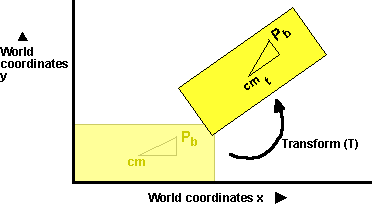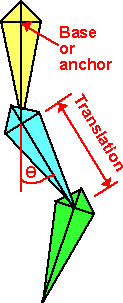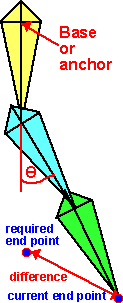3D Theory - Kinematics - Joints

Representing motion in 3D

To model motion of a body, we can use a transform (T) to transform each point relative to the body to a position in world coordinates.If we want to model both liniear and angular (rotational) motion then we need to use a 4x4 matrix to represent the transform.

If we only need to model rotation then, we can reduce this to either use a 3x3 matrix or a quaternion.

Modelling rotations in 3D is not always intuative. For instance, a rotation of 90 degrees about the x-axis followed by a rotation of 90 degrees about the y-axis is equivilant to a rotation of 90 degrees about the z-axis. More information about rotations.

Frameworks, Chains (or Skeletons)

A lot of mechanical objects in the real world consist of solid sections connected by joints. Examples of this might be:

• Creatures such as humans and animals.
• Car Suspension
• Robot arms
• Ropes, string and Chains.
• Etc.

Where you have something like a robot arm, each section of the arm can pivot, bend and/or rotate from the previous stage. These sections and joints are known as a 'chain', if we are talking about creatures then it could be referred to as a skeleton. Similarly in biological terms a movable section can be referred to as a bone, and the attachments between each bones as joints.

Motions of chains can be specified in terms of translations and rotations.

• Forward Kinematics - From the amounts of rotation and bending of each joint in an arm, for example, the position of the hand can be calculated.
• Inverse Kinematics - If the hand is moved, the rotation and bending of the arm is calculated, in accordance with the length and joint properties of each section of the arm.

Forward KinematicsThis Chain is represented here, without any information about its actual shape, what we are interested in is the relative positions of all the joints. The first link is the base (or "anchor point" in nautical terms) is the end of the chain which always has a fixed or known position. From there, each link can be rotated (possibly in different dimensions depending on the degree of freedom of the joint). The other important factor is the distance between the joints, this can be represented as a translation in a TransformGroup. This transform group can also hold the rotation of that link. Therefore this chain can be represented by a hierarchy of TransformGroups.

So Forward Kinematics allows one to find the position of the endpoint (and all the points in-between) relative to the base. This is easy to do, just multiply by the TransformGroup matrixes in turn.

Inverse Kinematics

For inverse kinematics (IK), the position of the end point is known, and we need to find the angles of the joints. This is a much harder problem, there may be many possible answers, or there may not be a set of angles that would reach to that point. Although this is hard to do, it is useful, for example, when posing a model it is useful to be able to drag the extremities with a mouse and have the arms/links position themselves appropriately.

There are two approaches to solving inverse kinematics:

• Analytical - requires a lot of trigonometry or matrix algebra
• Iterative - better if there are lots of links and degrees of freedom.

IK - Analytical approach

If there are only two or three links then it may be possible to solve it analytically. One possibly might be to draw out the arm with the angles shown on it, then solve for the angles using geometry, a good knowledge of trig identities would help here. The problem is that this is not really very general approach.

Another analytical approach is to represent each links rotation and translation by a matrix. The end point is then given by all these matrixes multiplied together, so we just need to solve this matrix equation. Then find what rotation each matrix represents.

There may be many solutions or there may not be any solutions. In other words there are lots of ways to reach to a given point, or it may be out of reach.

If there are many solutions, then you might need to apply additional constraints. For instance, human joints can only bend within certain limits.

IK - Iterative -approach

This is a more general approach for programming complex chains.Start off with the joints in any position, then move each of the joints in turn, so that each movement takes the endpoint toward the target. Starting with the joint nearest the end point, rotate the joint so that the current end point moves toward the required end point. Then do the same with the next joint toward the base and so on until the base is rotated. Then keep repeating this, until the end point is close enough to the required end point or if further iterations are not moving it closer to the required point. It may be possible to have a more realistic strategy than this, for instance, if I am using my arm to pick up an object then, if the object is a long way away, I will move the bigger joints in the arm, then as the hand gets closer the smaller joints of the hand are used for the fine adjustments. The angle of rotation for each joint is found by taking the dot product of the vectors from the joint to the current point and from the joint to the desired end point. Then taking the arcsin of this dot product. To find the sign of this angle (ie which direction to turn), take the cross product of these vectors and checking the sign of the Z element of the vector.

more on IK here

Human Animation

A human skeleton can be represented as a hierarchical set of joints, see following diagram from mpeg4This can be represented by a scenegraph tree of transform groups (see this extract from VRML h-anim spec, just a small section of human skeleton)

HumanoidRoot : sacrum
sacroiliac : pelvis
|   l_hip : l_thigh
|     l_knee : l_calf
|       l_ankle : l_hindfoot
|         l_subtalar : l_midproximal
|           l_midtarsal : l_middistal
|             l_metatarsal : l_forefoot
|   r_hip : r_thigh
|     r_knee : r_calf
|       r_ankle : r_hindfoot
|         r_subtalar :  r_midproximal
|           r_midtarsal : r_middistal
|             r_metatarsal : r_forefoot
vl5  : l5
vl4 : l4
vl3  : l3
vl2 : l2
vl1  : l1
vt12 : t12

<snip>...

This skeleton is then combined with a physical model of the human/creature to produce the animation

h-anim standards

 metadata block see also: Correspondence about this page Book Shop - Further reading. Where I can, I have put links to Amazon for books that are relevant to the subject, click on the appropriate country flag to get more details of the book or to buy it from them.Robots and Screw Theory: Applications of Kinematics and Statics to Robotics (Hardcover) - This is about the mechanics of robot arms such as those used such as those used in factory automation. Assumes a good knowledge of mechanics but the concepts of robot arms and screw theory are introduced gradually. The maths used is fairly conventional: scalars, vectors and matrices. Dual quaternions (or biquaternions as they call them) are only mentioned briefly. Commercial Software Shop Where I can, I have put links to Amazon for commercial software, not directly related to the software project, but related to the subject being discussed, click on the appropriate country flag to get more details of the software or to buy it from them.LEGO Mindstorms NXT - Allows you to build a robot and program it from a PC or Mac. Contains an Intelligent Brick with 32-bit microprocessor, memory and FLASH, 3 Interactive Servo motors with built-in rotation sensors, sound sensor, ultrasonic sensor, touch sensor, light sensor, USB 2.0 and Bluetooth support. Icon-based drag-and-drop program "building" environment.

This site may have errors. Don't use for critical systems.